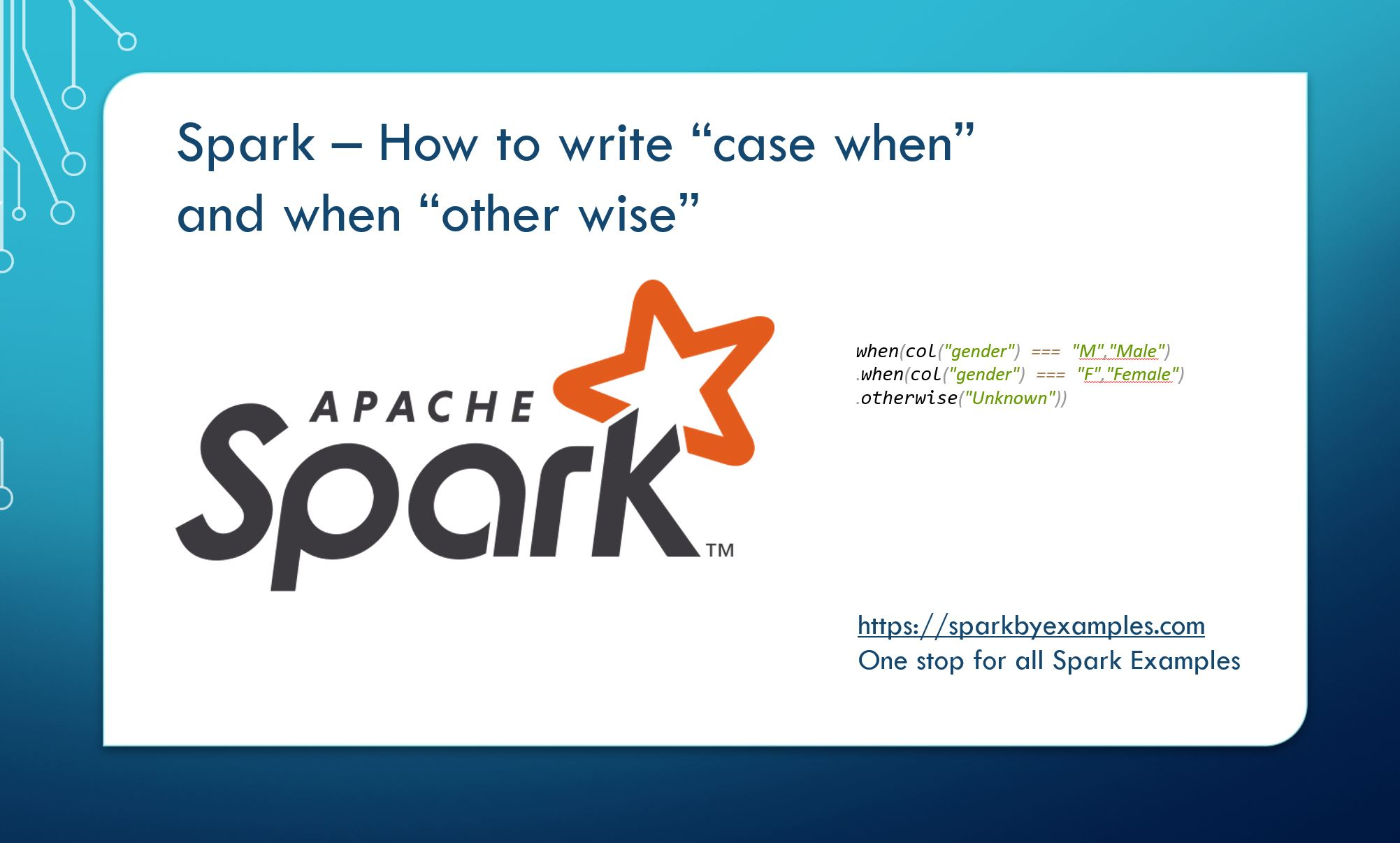# Spark SQL “case when” and “when otherwise”

Like SQL `"case when"` statement and “`Swith"`, `"if then else"` statement from popular programming languages, Spark SQL Dataframe also supports similar syntax using “`when otherwise`” or we can also use “`case when`” statement. So let’s see an example on how to check for multiple conditions and replicate SQL CASE statement.

First Let’s do the imports that are needed and create spark context and DataFrame.

``````
import org.apache.spark.sql.functions.{when, _}
val spark: SparkSession = SparkSession.builder()
.master("local")
.appName("SparkByExamples.com")
.getOrCreate()

import spark.sqlContext.implicits._
val data = List(("James","","Smith","36636","M",60000),
("Michael","Rose","","40288","M",70000),
("Robert","","Williams","42114","",400000),
("Maria","Anne","Jones","39192","F",500000),
("Jen","Mary","Brown","","F",0))

val cols = Seq("first_name","middle_name","last_name","dob","gender","salary")
val df = spark.createDataFrame(data).toDF(cols:_*)
``````

### 1. Using “when otherwise” on Spark DataFrame.

`when` is a Spark function, so to use it first we should import using `import org.apache.spark.sql.functions.when` before. Above code snippet replaces the value of gender with new derived value. when value not qualified with the condition, we are assigning “Unknown” as value.

``````
val df2 = df.withColumn("new_gender", when(col("gender") === "M","Male")
.when(col("gender") === "F","Female")
.otherwise("Unknown"))
``````

`when` can also be used on Spark SQL select statement.

``````
val df4 = df.select(col("*"), when(col("gender") === "M","Male")
.when(col("gender") === "F","Female")
.otherwise("Unknown").alias("new_gender"))
``````

### 2. Using “case when” on Spark DataFrame.

Similar to SQL syntax, we could use “case when” with expression `expr()` .

``````
val df3 = df.withColumn("new_gender",
expr("case when gender = 'M' then 'Male' " +
"when gender = 'F' then 'Female' " +
"else 'Unknown' end"))
``````

Using within SQL select.

``````
val df4 = df.select(col("*"),
expr("case when gender = 'M' then 'Male' " +
"when gender = 'F' then 'Female' " +
"else 'Unknown' end").alias("new_gender"))
``````

### 3. Using && and || operator

We can also use and (&&) or (||) within when function. To explain this I will use a new set of data to make it simple.

``````
(66, "a", "4"), (67, "a", "0"), (70, "b", "4"), (71, "d", "4"
)).toDF("id", "code", "amt")
when(col("code") === "a" || col("code") === "d", "A")
.when(col("code") === "b" && col("amt") === "4", "B")
.otherwise("A1"))
.show()
``````

Output:

``````
+---+----+---+----------+
| id|code|amt|new_column|
+---+----+---+----------+
| 66|   a|  4|         A|
| 67|   a|  0|         A|
| 70|   b|  4|         B|
| 71|   d|  4|         A|
+---+----+---+----------+
``````

#### Conclusion:

In this article, we have learned how to use spark “`case when`” using `expr()` function and “`when otherwise`” function on Dataframe also, we’ve learned how to use these functions with && and || logical operators. I hope you like this article.

Happy Learning !!

### NNK

SparkByExamples.com is a Big Data and Spark examples community page, all examples are simple and easy to understand and well tested in our development environment Read more ..

### This Post Has 8 Comments

1.Hi! When to use expr and which all functions can be used inside expr? Also, all the built in functions can be used on dataframes?

2.nausheen aafreen

I really love the contents. They are self explanatory. I am very happy that you have shared so much to learn ,for people like me who have no idea about spark.
You are amazing <3

1.NNK

Hi Nausheen, Thanks for your comments. I am happy it serving the purpose.

3.Anonymous

good article for experienced folks as well

4.Anonymous

how to use 3. Using && and || operator in using string expressions

5.Naveen V

Very useful information for beginners to practice.
6.1.